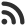Feed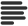Articles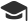Tutorials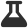Lab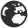CompaniesLeaderboard
 DG Student at BIT Mesra Aug. 21, 2020, noon ⋅ 454 views

# Deloitte Placement paper Logical Reasoning Questions with answer

1. If a man goes 20 kms to the South and then 15 kms to the east. At what distance he is from the starting position?

A. 20 kms

B. 15 kms

C. 10 kms

D. 25 kms

Ans. D

2. If South-East becomes North and North-East becomes West and all the rest directions are changed in the same manner then what will be the direction for West?

A. South

B. South-West

C. South-East

D. North-East

Ans. C

3. Find the next number in the series 2, 3, 6, 18, 108, …..

A. 1984

B. 1944

C. 2044

D. None of these

Ans. B

4. Choose the odd one

A. Keyboard

B. Mouse

C. Printer

D. Scanner

Ans. C

5. Sumit is 8th rank ahead of Ravi in a class of 45. If Ravi rank from the bottom is 19th then find the rank of Sumanth from the beginning?

A. 17

B. 18

C. 19

D. 20

Ans. C

6. Thermometer: Temperature :: Barometer:?

A. Stress

B. Pressure

C. Strain

D. Force

E. None of these

Ans. B

7. How many students did secure First class in a class of 50 students?
I. Double the number of first-class students secured second class and all the remaining
students failed
II. Number of students failed was twice the number of first class students in the class

A. Only I

B. Only II

C. Both I and II

D. Either I or II

E. Neither I or II

Ans. C

8. Four of the following five are alike in a certain way and so form a group. Which is the one that does not belong to that group?

A. Jackal

B. Horse

C. Cat

D. Dog

E. Wolf

Ans. B

9. Four of the following five are alike in a certain way and so form a group. Which is the one that does not belong to that group?

A. BCDF

B. KJNL

C. RSTU

D. WXYZ

E. FGHP

Ans. C

10. 522 1235 2661 4800 7652 11217 ?

A. 15495

B. 16208

C. 14782

D. 16921

Ans. A

11. I. 15 6 21
II. P 3 27
If ‘P’ is the resultant of the first row, what will be the resultant of the second row?

A. 27

B. 60

C. 58

D. 82

Ans. B

12. I. 51 17 35
II. 14 15 R
If ‘R’ is the resultant of the first row, what will be the sum of the resultants of first and second row?

A. 31

B. – 3

C. 29

D. 3

Ans. C

13. I. 12 36 15
II. 18 Q 10
If ‘Q’ is the resultant of the first row, what will be the resultant of the second row ?

A. 85

B. 101

C. 69

D. None of the above

Ans. D

14. In a row of 21 boys when akash has shifted four places to the right, he becomes 12th from the left enD. What was the akash earlier position from the right end.

A. 10

B. 11

C. 13

D. 14

Ans. D

Q15. Thermometer: Temperature :: Barometer:?

A. Strain

B. Force

C. Stress

D. Pressure

Ans. D

Events

Nov. 28, 2018, 5:30 p.m.

Python from zero to hero

place Delhi

Aug. 13, 2018, 5:30 p.m.

Python from zero to hero

place Bangalore ( HackersFriend office BTM Layout)